What is Scanning? Scanning is the process of consuming characters. Maybe ASCII or Unicode, and looking for patterns that indicate groupings. The letters 'i' and 'f' are two characters, but together, they are 'if', a C keyword used to branch on a condition. If you have ever used regular expressions, you know how useful they are. You can use 'grep' to find text in a file that matches the regular expression. If you haven't use regular expressions, pause here and learn how to use them. The rest of this document assumes that you understand how to use regular expressions. Scanning, as LALRLib does it, uses regular expressions. Instead of using one like grep does, a scanner starts out with a comprehensive set of candidate regular expressions, each associated with a token type. As input characters are consumed, regular expressions that don't match fall away. The last one to still match is the one that ultimately decides which token type it matched.

## Conventions Used Here

Note that in the following, I'll use the shorthand 'regex' to mean 'regular expression'. Regexes will be shown inside two slashes that are not part of the regular expression. Strings to run against the regular expression will be in either double quotes or double quote prefixed with an @ sign. I use C# conventions, i.e. strings in "" will have its elements properly escaped and strings in @"" will use a double set of quotation marks to represent a single pair but everything else will be verbatim. For example, these two strings are the same: They both evaluate to: start\"\end

## States

When using a lexical scanner, you may find yourself wanting to match different sets of regular expressions depending on some state. In languages with C like comments, it can be useful to enter a COMMENT state when the /* is seen and leave that state when the */ is seen. That way, the scanner doesn't try to match tokens inside the comment.

## Turning Regular Expressions into a Non-deterministic Finite Automata (NFA)

This section will explain how to take regular expressions and turn them into Nondeterministic Finite Automata. Before explaining, these conventions will be used in the explanations. States
• Triangular state: a start state
• Square state: an accept state for a matched regular expression
• Round state: a valid intermediate state but not matched
Transitions
• Lambda (λ): a transition that is automatic and instantaneous.
• Any other character: a transition
• Non-existent transitions: error condition or accept
The fundamental operation of a state machine is that while the machine is in a active state, inputs cause transitions from that state to another as they are consumed. The inputs are simply the characters of an input string. If there is a transition to another state for that input, then the machine activates that state. If there is no transition, then there are two possibilities. First, if the state is an accept state, then the machine has matched whatever pattern the state accepts and it returns the associated token type. The other case is if the state is not an accept state. This is an error. It means that scanning has failed to match a valid input string. What's the N in NFA? What does it mean that it's "non-deterministic"? In an NFA, there may be more than one active state at any one time if a single transition goes to multiple states. When more than one state is active, if a transition is an error but there are still other active states, then the matching continues with the set of states that are still active. It continues until the final active state goes out. In fact it is possible that there are multiple active states and all go out simultaneously. In that case, the machine designer must decide which accept to match. In Figure 2 A: NFA for /if/and /in/, 'i' coming out of the start state 0 goes to both states 1 and 2. Both are active. The 'n' ends the matching of "if" because there is no transition from state 1 on 'n'. But state 2 goes to state 4 on 'n'. Finally, a space would cause matching to terminate as there is no transition on state 4, and since it's in an accept state, it means that "in" has been matched.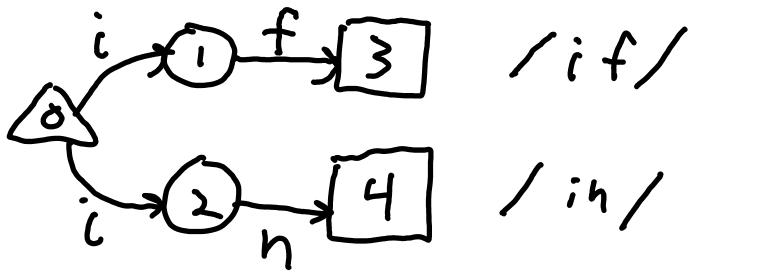Figure 2 A: NFA for /if/and /in/ showing transition to two NFA states on 'i' There is a special transition called a lambda (λ) transition. When in an active state that has lambda transitions to other states, the machine also activates those states immediately and automatically. This cascades until there are no more unfollowed lambda transitions. Note that the transitions are directional, so if state m has a lambda transition to state n, then if state m is an active state, state n is then also an active state. But, state n being active doesn't make state m active. Lambda transitions are important in the construction of NFA state machines. They will be shown with a 'λ' by the transition. Now that the state machine has been described, let's look at how regular expressions are converted to NFA state machines. This section will start simple and introduce concepts as it goes. There will be both graphical representations and pseudo code algorithms. Every NFA starts with a start state. As we build the NFA, we keep track of the 'current' state and previous current state which will be called 'prev'. New states are created as needed and transitions from existing states are connected to the new states. The 'current' and 'prev' are then updated. Note that 'prev' may be null as there are cases where there is no previous state.

### Temporary NFA Start Routine

The following algorithm describes a plausible way to start the NFA state machine. It will be refined later, but for now, it's a good way to get things started. Figure 1 A shows the NFA at the start.Figure 2 B: A reasonable tentative was to start an NFA.

## Character Matching

The simplest example is simple character matching. A new state is made, and a transition is created from 'current' to the new state on the particular character. Then 'prev' gets 'current' and 'current' is set to the new state. Figure 1 B shows /LALR/ NFA state. This is the result of 4 additions to the start state, one for each letter. The algorithm for adding a new state to the state machine is: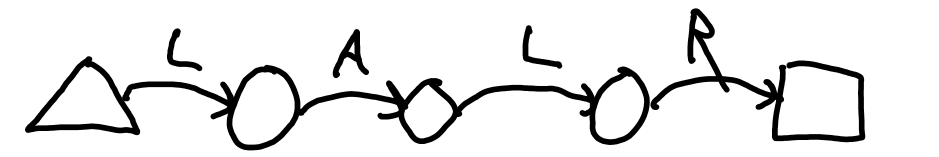Figure 2 C: NFA for /LALR/.

#### . (Dot)

The dot simply causes a transition on every character except '\n' (newline). In Figure 1 C, see the dot as /A.C/ consumes "ABC" or "A#C" or "AaC" or even "A.C" or "A C". It does not match "A C" where there is a newline between A and C.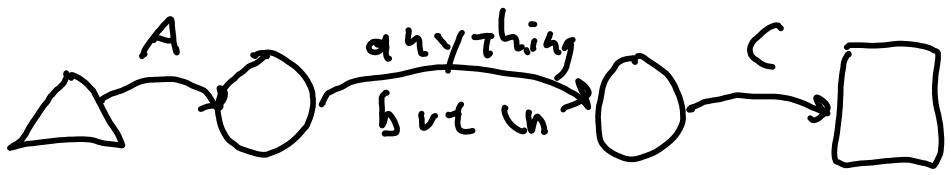Figure 2 D: NFA for /A.C/.

#### () and |

The next item to consider is the () and |. As with the anchors, these have special states. When the '(' is encountered, two NFA states are created: initial and final. Then a third state is created and a lambda is run from initial to it. This third state becomes the current state, i.e. the one to build off of. When | is encountered, a lambda transition is created from the current state to final, a new state is created, and a lambda is run from initial to this new state. When ')' is encountered, a lambda transition is set up from the current state to final. Then the final state becomes the new current state and the initial state becomes the new 'prev'. Figure 1 K shows the complete NFA for /(SEA|YVR|PDX)/. The states on the ends are the initial and final. The concept nests. Figure 1 L shows /(SEA|YVR|PDX(MKE|ORD))/.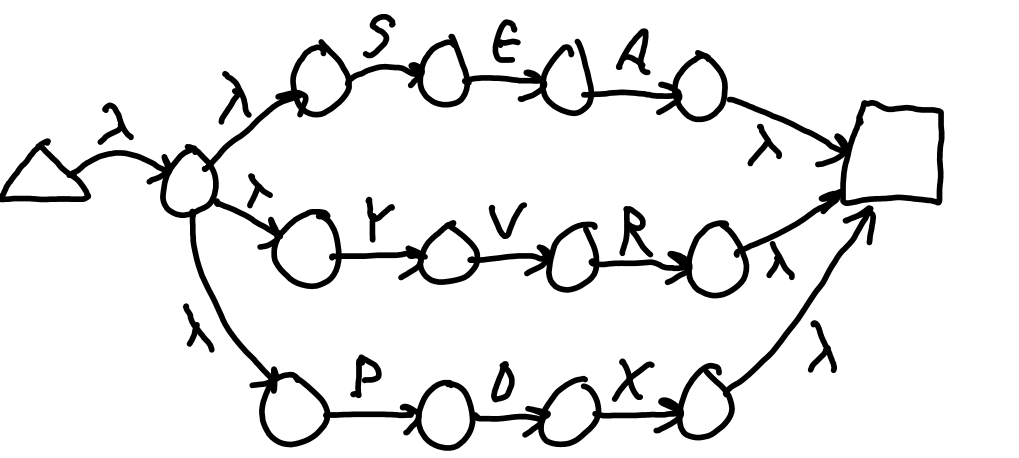Figure 2 L: NFA for /(SEA|YVR|PDX)/.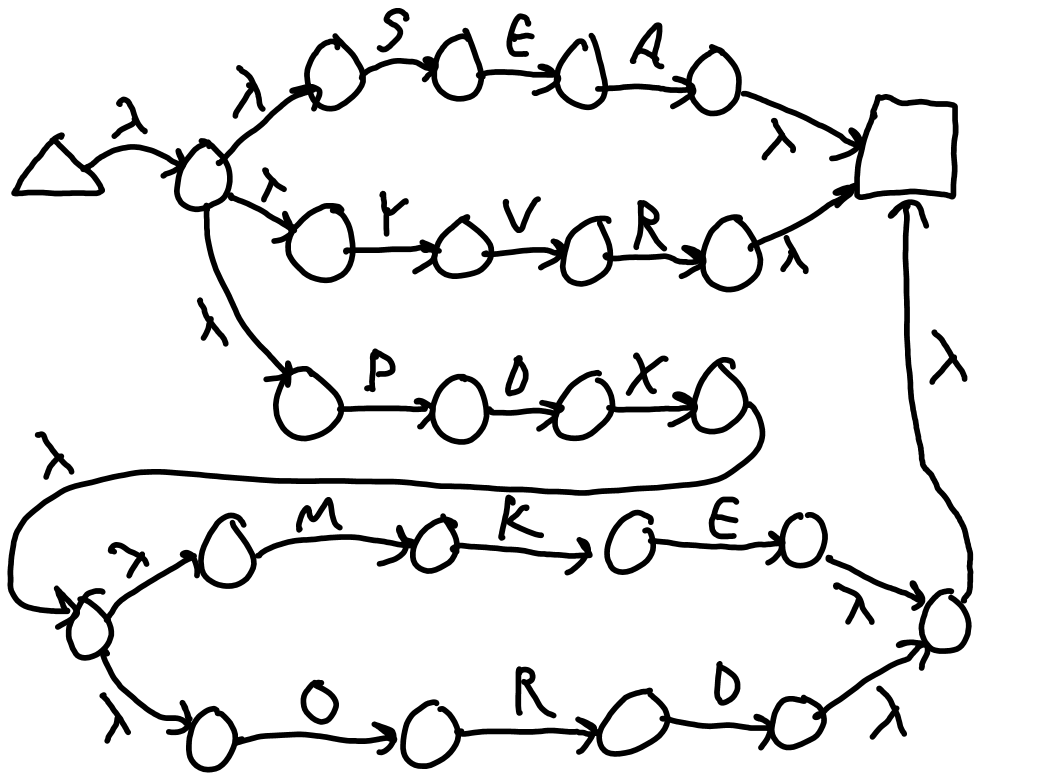Figure 2 M: NFA for /(SEA|YVR|PDX(MKE|ORD))/. The algorithm for processing ( is shown: The algorithm for processing ) is shown: Finally, the algorithm for processing | is shown:

#### Top Level Implied ()

Note the // that go around the regex are sort of like implicit parentheses. This is because the | operator must work at the outer most level. That is, /MKE|MSP|ORD/ is equivalent to /(MKE|MSP|ORD)/. That means that every regex NFA has an initial state and final state just inside the anchor states. Thus, our /LALR/ will actually look like Figure 2 N which is like as if it were /(LALR)/.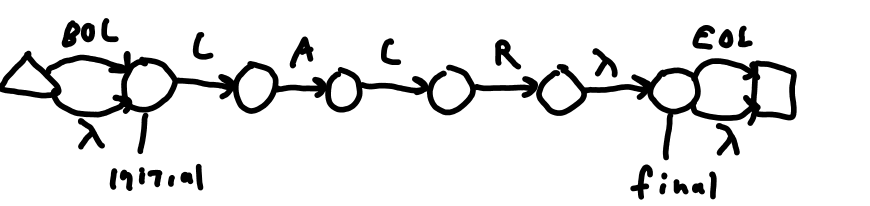Figure 2 N: Initial and final states from top level Now that the concept of the current pointer has been established, it is time to start explaining how ?, +, and * work. This is where the 'prev' pointer, i.e. the previous current pointer starts to come in. So for these next three patterns, pay attention to the previous and current states.

#### ?

The question mark skips over the item whose state was just created. Consider /AB?C/ which matches "AC" or "ABC". When the regex interprets the '?' having 'current' at the state 2 and 'prev' at state 1, it creates a new state (3) and runs a lambda from both 'prev' and 'current' to this new state. Then 'prev' is nulled and the new state is made current. Now the state 4 transitions on C from 3 to 4. The result is that when state 1 is active, state 3 is necessarily active. So a transition on C from state 1 goes straight to state 4. Alternatively, state 1 can transfer on 'B' to state 2 which still has state 3 active. The 'C' then transitions to state 4. So the state machine matches both "AC" and "ABC". See the progression in Figure 1 M.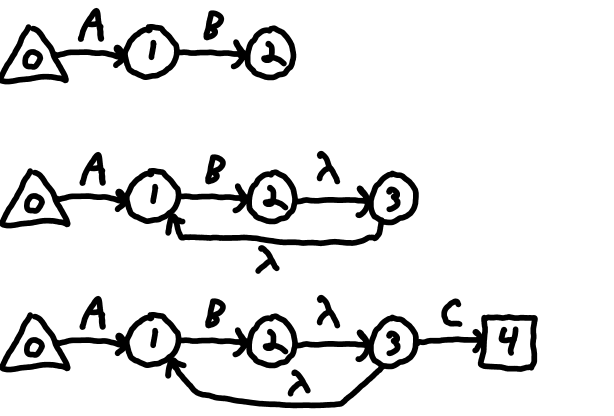Figure 2 ONFA for /AB?C/ The algorithm is:

#### +

The plus causes the transition between 'prev' and 'curren' to be matched once, and then matched again indefinitely. Again, 'prev' points to state 1 and 'current' to state 2. A new state is made (3), a lambda transition from 'current' to the new state is created and a lambda from 'current' to 'prev' is made. Then 'prev' is nulled and 'current' is set to the new state. So, for /AB+C/, when in state 2, state 3 is also active and state 1 is active after all the cascading is done. The 'B' must be matched to get to state 2, but after that, B can be matched indefinitely, until the C moves onto state 4 which is the accept state.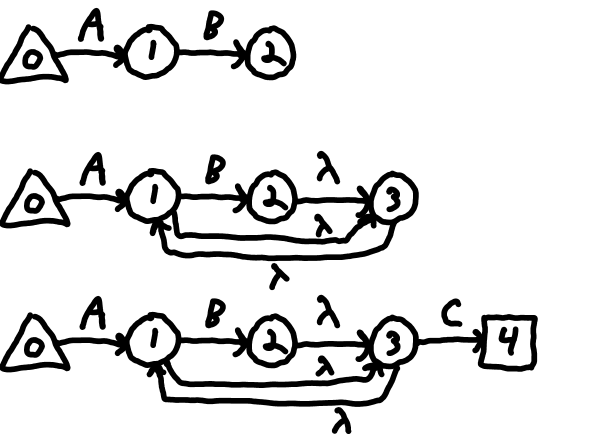Figure 2 P: NFA for /AB+C/ The algorithm is:

#### *

Finally, the * is like a combination of ? and +. Figure 1 N shows /AB*C/. Again, 'prev' points to state 1 and 'current' to state 2. A new state is made (3), a lambda transition from 'current' to the new state is created and a lambda from 'current' to 'prev' is made. In order to skip over the item, a lambda transition from 'prev' to the new state is also set up. Then 'prev' in nulled and 'current' is set to the new state. So, when the 'A' takes the state machine to state 1, state 3 is also active so a 'C' will transition to state 4. Or a 'B' will make state 2 active which also makes state 1 and state 3 active. From state 1, again, 'B' can be matched and from state 3, 'C' can be matched.Figure 2 Q: NFA for /AB*C/. The algorithm is:

#### Other Regex Techniques

This is all the LALRLib does. Some regex engines use \ to set up groupings like digits. Also, there are a large set of Unicode code that are not supported.

#### Determining the Accept State

When the regex is converted into an NFA, there state which is current is the "accept state". In fact every state which can reach it via lambda transitions are also in that same accept state, but let's defer that problem since the next step doesn't need us to figure out at this point which states other than 'current' are also accept states. The accept ID is typically a number associated with the regex string. In the case of LALRLib, it is the index of the token type array with the regex.

#### Merging NFAs

Finally, all of these NFAs have to be merged into one big NFA so that we can start with one state and reach all possible accept states. To merge all of the NFAs for each regex, a new state is created. This is the true root state. The root state has a lambda transition to the first state of each NFA. Consider this set of regexes: Figure 1 O shows the whole NFA. This has the () implied by //, an anchor, and several transitions on 'I'. This will be used in the next section where it will be turned into a DFA.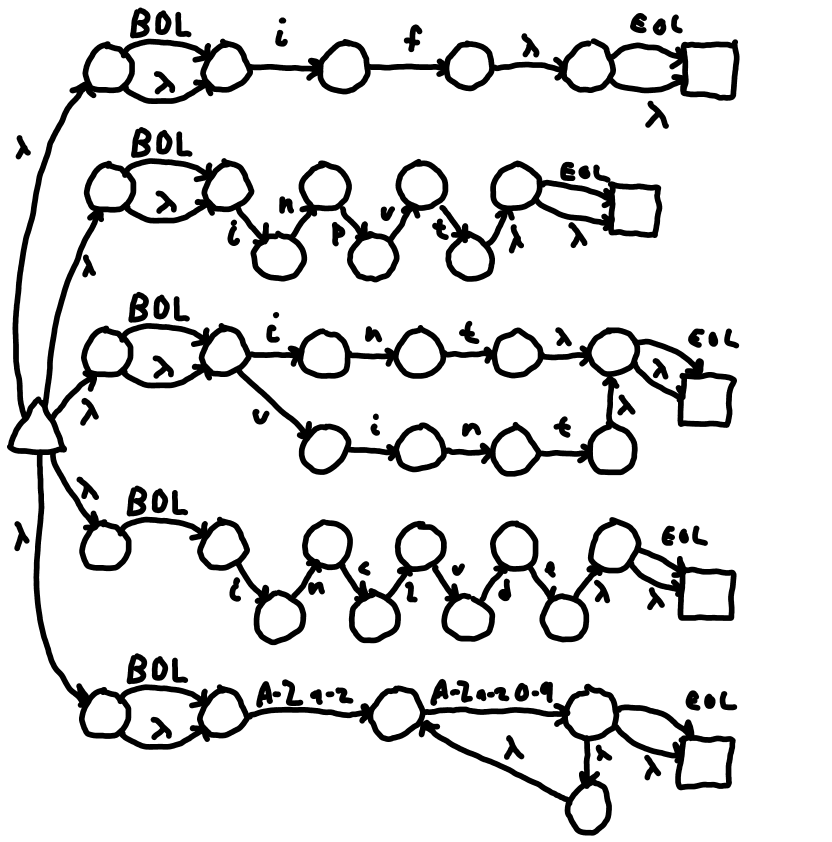Figure 2 R: A merged NFA. The algorithm for merging is:

#### Turning a Non-deterministic Finite Automata into a Deterministic Finite Automata

While an NFA is useable by following a set of valid states and transitioning on each one keeping track of which NFA states are still active, there is a better solution. Convert the NFA into a Deterministic Finite Automata (DFA). This essentially does exactly that, but only once at the beginning when the conversion is done—not while actually using the NFA state machine against real inputs. A DFA state is really just the list of all NFA states that are currently active. The first state is the DFA state that corresponds to NFA state 0—the root state. Remember, in an NFA with lambda transitions, if an NFA state is active, so are all other states reachable by following lambda transitions. So in fact, the first DFA state will be include, potentially, a lot of NFA states. So looking at Figure 1 P, the set of all NFA states reachable from NFA state 0 is surrounded by the thin line.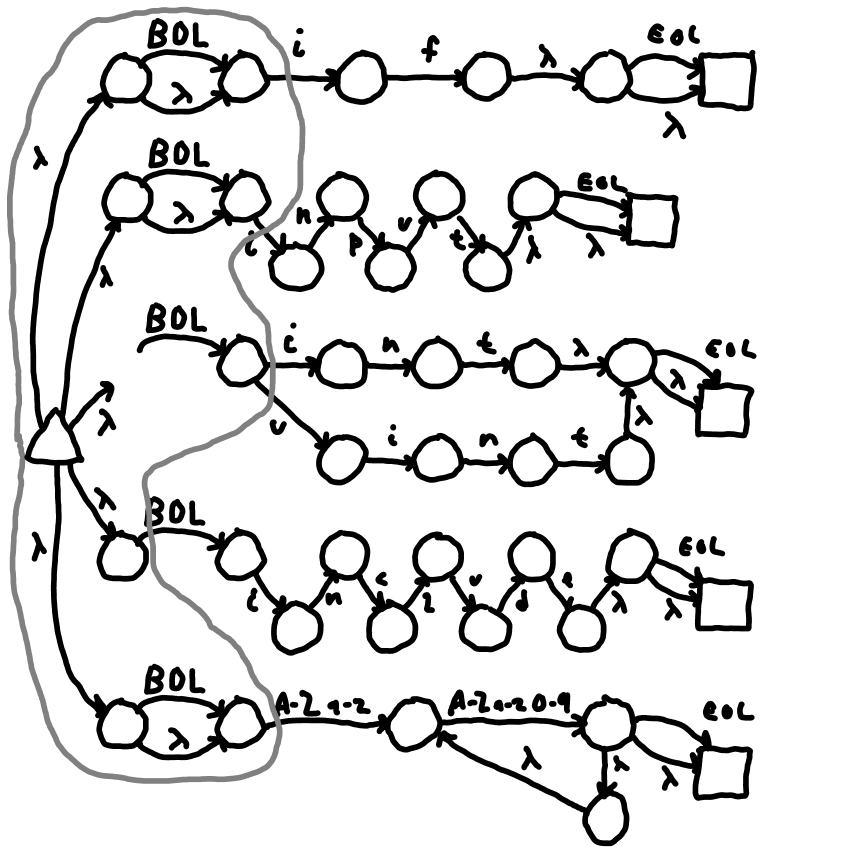Figure 2 S: NFA with DFA0 states indicated. Now let's make DFA states 1. Consider the transition BOL (beginning of line). From all active NFA states in DFA state 0, there are BOL transitions to the states shown in Figure 1 Q. Note NFA state 0 is longer part of DFA 1. And the /^include/ NFA is started.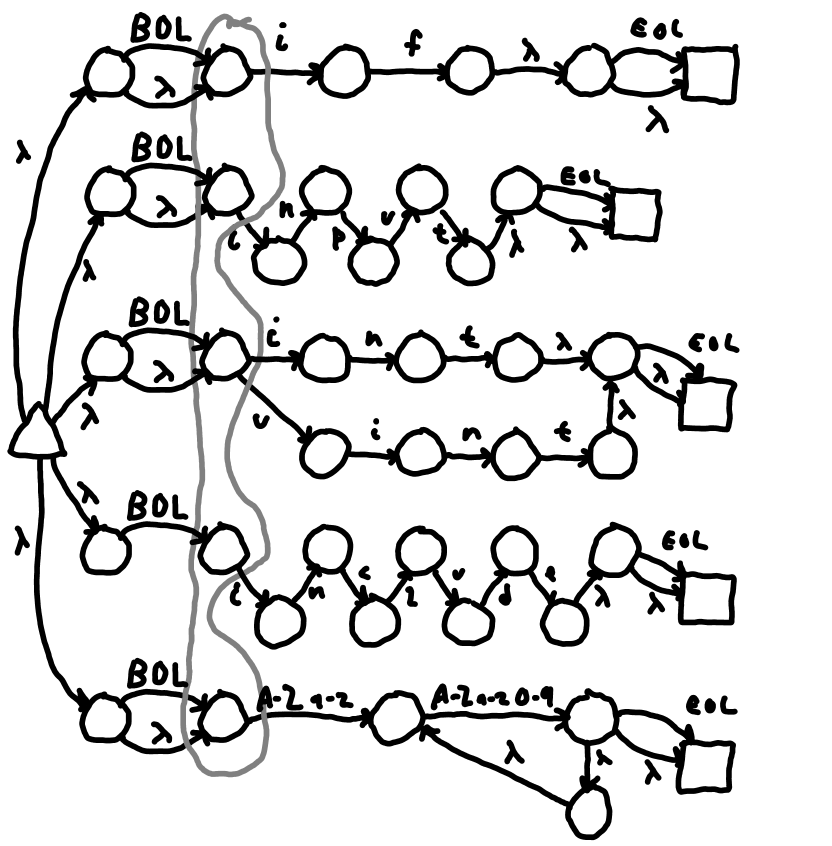Figure 2 T: DFA 1 with NFA states active after BOL transition from DFA0. Two more examples for DFA 2 and DFA 3 are the transitions on 'i' from DFA 0 and DFA 1 respectively. DFA 0 transitions to DFA 2 on 'i' and notice that it cannot get to /^include/ as seen in diagram 20. While DFA 3 transitions from DFA 1 on 'i' and can match /^include/.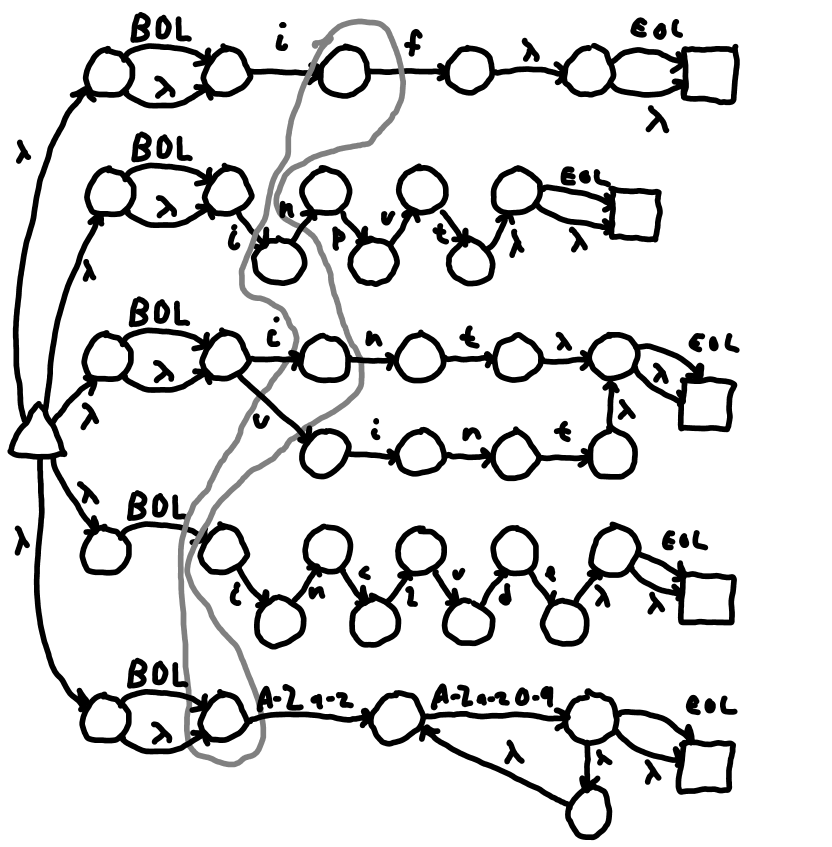Figure 2 U: DFA 2 showing transition on 'i' from DFA state 0.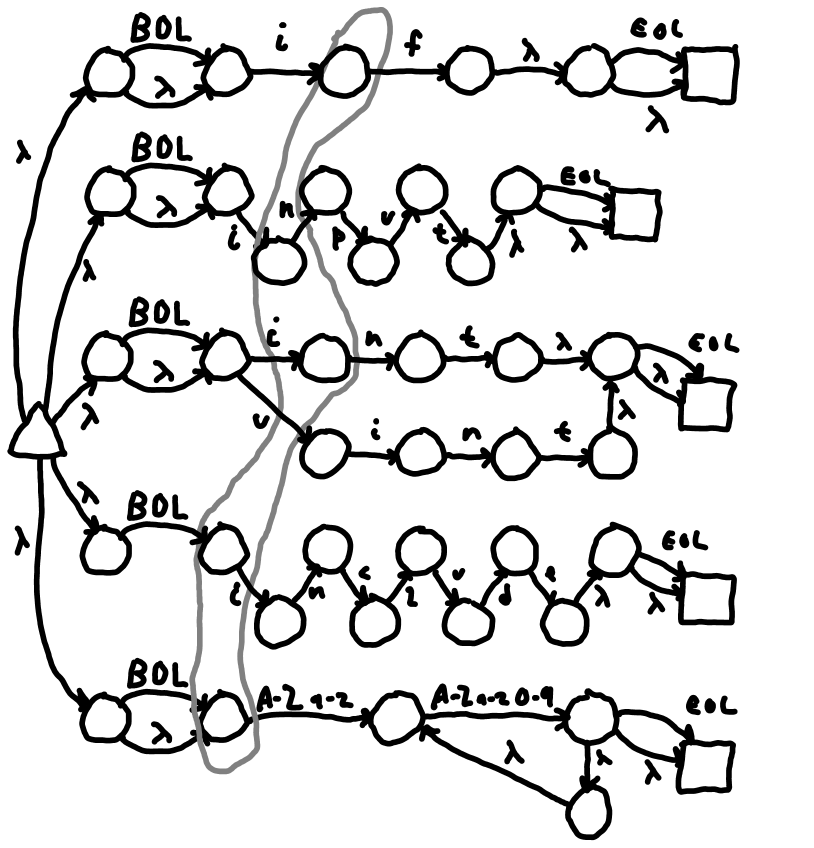Figure 2 V: DFA 3 showing states active after DFA0 sees BOL and then 'i'. This is done for every transition for every added DFA state. The set of all NFA states active are determined. If there is already a DFA state containing an identical set of NFA states, that is used. If not, a new DFA state is created. This is done until all transitions on all DFA states have been considered. The final step in the conversion of NFA to DFA is to determine the accept states. If there are no NFA states that are accept states, then the DFA is not an accept state. So a nul transition results in an error. If exactly one NFA state is an accept state, the DFA is an accept state that accepts what the NFA accepts. There are, however cases where there can be more than one accept state. In the regexes above, "if" will match both /if/ and /[A-Za-z_][A-Za-z0-9_]+/. The method used by the solution here is to assume that the regexes are in order from more specific to less specific. Thus, when there are more than one regexes that can be accepted, the one with the lowest valid accept ID wins. Thus 'if' will be matched by /if/. If I had placed /[A-Za-z_][A-Za-z0-9_]+/ first, then it would match 'if', and the /if/ regex would never even be matched. The algorithm for getting the complete set of NFAstates reachable via λ transitions from an original set is: The algorithm for creating a complete DFA state from a set of one or more NFA states is: The method of creating or getting the DFA is:

#### Creating Scan Tables

It is possible to group sets of regular expressions together into scan states. There will be one root NFA for each scan state which goes to all the regex NFAs for that state. This is why LALRLib has regexes are passed to the compiler in a dictionary with the array index as a key rather than as an array. Scan states are manipulated in user provided code called when a new token is processed during scanning. An example is C style comments. When a @"/*" is matched. The scanner can be switched into a state where it only seeks the end of the comment. This is actually a fairly difficult problem as most the obvious solutions fail to match the end of the comment under certain conditions. One solution is to seek the following regexes: The first regex eats asterisks not immediately followed by a slash one by one which is not necessarily efficient, but it works. The second eats everything up to an asterisk. The last one should revert the state back from the comment state. Now that we have a DFA for each scan state, we can make a scan table for each scan state. The scan table is a big 2 dimensional array where one axis is each character that the DFA can transition on and the other axis is the DFA number. Each entry contains a new DFA (or -1 if no transition) and a regex index accept ID to indicate a successful match (or -1 if no match). The algorithm for creating a scan table from a DFA array is:

# Driving the Scan Table

Once the scan table is created, it is necessary to drive it based on an input stream. The stream may be a string or a file. In either case, the driver has to keep track of line location so that it can generate the BOL and EOL characters. It should also produce an EOF character and λ for which there is no transition in order to force the accept of the very last token which may be in an accept state but won't be considered a match until the failure to transition. Driving the table is easy. There is a current DFA state, a way in indicate the characters matched so far, and the input character. A new match attempt always starts of DFA 0. If there is a valid transition on the input character for the DFA, the DFA state number changes to that and the character is remembered (either by explicitly storing it or by updating pointers). In LALRLib, it is saved in a StringBuilder. Saving the beginning and end pointers or indices is another option. This operation continues until there in an invalid transition out of the current state. If that state is an accept state, the match ID and the string are returned. If there is no accept ID, then an error is returned because that string is unscannable. The algorithm is:

### Non-Matching Regexes

It is possible to make non-matching terminals in LALRLib. These will start a token, but the processing will cancel the token. It is still able to do process scan states or manipulate the context object.